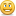###### 9 Repliesstop();

speed = 10;

initial_width = 60;

target_width = 678;

sp = rect1._x;

this.createEmptyMovieClip("emptymc", 0);

emptymc.onEnterFrame = function() {

for (i=1; i

if (_root.hit.hitTest(_root._xmouse, _root._ymouse, true)) {

if (_root["rect"+i].hitTest(_root._xmouse, _root._ymouse, true)) {

new_x = (_root.sp-(60*(i-1)))-rect1._x;

rect1._x += new_x/speed;

n_width = target_width-_root["rect"+i]._width;

_root["rect"+i]._width += n_width/speed;

} else {

n_width2 = initial_width-_root["rect"+i]._width;

_root["rect"+i]._width += n_width2/speed;

}

} else {

new_x = _root.sp-rect1._x;

rect1._x += new_x/(speed+50);

n_width2 = initial_width-_root["rect"+i]._width;

_root["rect"+i]._width += n_width2/(speed-1);

}

_root["rect"+(i+1)]._x = _root["rect"+i]._x+_root["rect"+i]._width;

_root["movie"+i]._x = _root["rect"+i]._x-1;

}

};

...a colleague helped me to see that the problem might be with the first 'if' line:

if (_root.hit.hitTest(_root._xmouse, _root._ymouse, true)) {

as the item hit is a movie clip that is used multiple times on the stage. Again, works fine standalone, but not so much in Presenter.Oh, well I already see some potential issuesYou're using _root to control positioning:-) That's a no-no cuz it conflicts with Presenter's player coding which also references _root. Here's more info Flash best practices in Presenter 09.

Is that your code? Can you modify to make the references relative instead of absolute?